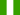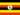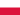48-hour FLASH SALE: Get 30% Off All Certificates and Diplomas! Hurry, sale ends on Thursday, 12th December 2019.

All Modules View all topics Close topics
Module 1: Understanding Fixed, Variable and Marginal Costs in Economics

# Introducing Fixed, Variable, and Marginal Costs

• Study Notes
• Discuss This TopicAmah K. 1 0 Fixed cost is the cost that remains constant when the firm is in a short run period. Total cost is the overall cost incurred on a firm. Marginal cost is the change in total cost divided by change in output or quantity.Mohamed Huseein A. 0 0 Explain fixed costKaram A. 0 0 Fixed cost is the cost that remains constant when the firm is in a short run period. Total cost is the overall cost incurred on a firm. Marginal cost is the change in total cost divided by change in output or quantity.
• Text Version